# Clustering in Machine Learning

In this page we will learn Clustering in Machine Learning, What is Clustering in Machine Learning?, Types of Clustering Methods, Partitioning Clustering, Density-Based Clustering, Distribution Model-Based Clustering, Hierarchical Clustering, Fuzzy Clustering, Clustering Algorithms, Applications of Clustering.

## What is Clustering in Machine Learning?

Clustering, often known as cluster analysis, is a machine learning technique that groups unlabeled data into groups. It can be defined as follows: "A way of grouping the data points into different clusters, consisting of similar data points. The objects with the possible similarities remain in a group that has less or no similarities with another group."

It accomplishes this by identifying comparable patterns in the unlabeled dataset, such as shape, size, color, and activity, and categorizing them according to the presence or absence of those patterns.

It is an unsupervised learning method, which means the algorithm receives no supervision and works with an unlabeled dataset.

Following the application of this clustering technique, each cluster or group is given a cluster-ID, which can be used by ML systems to facilitate the processing of huge and complicated datasets.

The clustering technique is commonly used for statistical data analysis.

Note: clustering is similar to classification, but the distinction is the sort of dataset we're working with. We work with the labeled data set in classification, whereas we work with the unlabeled dataset in clustering.

Example: lets understand the clustering technique with real world example of mall: When we go to a shopping center, we notice that items that are used in the same way are grouped together. T-shirts, for example, are arranged in one section and pants in another; similarly, in the vegetable section, apples, bananas, mangoes, and other fruits and vegetables are grouped in separate sections so that we can easily discover what we're looking for. The clustering process operates in a similar manner. Clustering can also take the form of grouping materials by topic.

The clustering technique can be applied to a wide range of jobs. The following are some of the most common applications of this technique:

• Market Segmentation
• Statistical data analysis
• Social network analysis
• Image segmentation
• Anomaly detection, etc.

Apart from these basic applications, Amazon uses it in its recommendation system to make product recommendations based on previous product searches. Netflix also employs this technology to suggest movies and web series to its subscribers based on their viewing habits.

The clustering algorithm is depicted in the diagram below. The many fruits are classified into multiple groups with comparable qualities, as can be shown.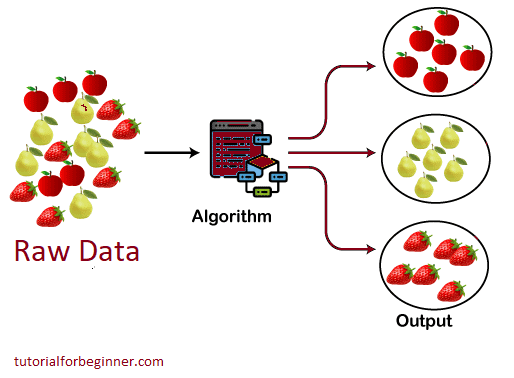## Types of Clustering Methods

Hard clustering (datapoints belong to just one group) and Soft clustering (datapoints belong to several groups) are the two types of clustering procedures (data points can belong to another group also). However, there are a variety of alternative Clustering techniques. The following are the most common clustering techniques used in machine learning:

1. Partitioning Clustering
2. Density-Based Clustering
3. Distribution Model-Based Clustering
4. Hierarchical Clustering
5. Fuzzy Clustering

## Partitioning Clustering

It's a clustering technique that splits data into non-hierarchical groupings. The centroid-based technique is another name for it. The K-Means Clustering technique is the most prominent example of partitioning clustering.

The dataset is partitioned into a collection of k groups in this type, with K indicating the number of pre-defined groups. The cluster center is designed so that the distance between data points in one cluster is the shortest possible when compared to another cluster centroid.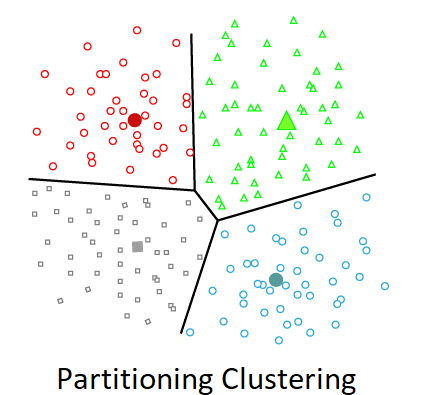## Density-Based Clustering

The density-based clustering method joins dense areas into clusters, resulting in arbitrary shaped distributions as long as the dense region can be linked. This program accomplishes this by finding several clusters in the dataset and connecting high-density areas into clusters. In data space, sparser zones separate dense areas from each other.

If the dataset includes varied densities and large dimensions, these algorithms may have trouble grouping the data points.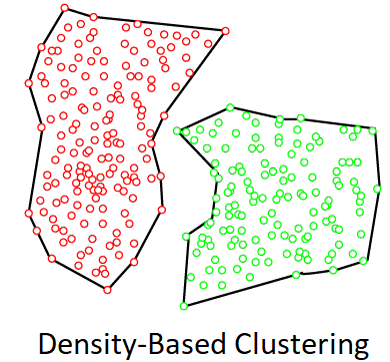## Distribution Model-Based Clustering

The data is separated based on the probability of how a dataset conforms to a certain distribution in the distribution model-based clustering method. The grouping is accomplished by assuming some distributions, the most popular of which is the Gaussian Distribution.

The Expectation-Maximization Clustering algorithm, which employs Gaussian Mixture Models, is an example of this type (GMM).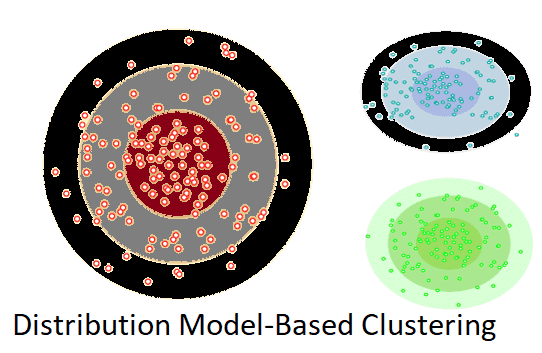## Hierarchical Clustering

Because there is no need to pre-specify the number of clusters to be produced, hierarchical clustering can be utilized as an alternative to partitioned clustering. The dataset is separated into clusters in this technique, which results in a tree-like structure known as a dendrogram. By pruning the tree at the proper level, you can select the observations or any number of clusters. The Agglomerative Hierarchical algorithm is the most common example of this strategy.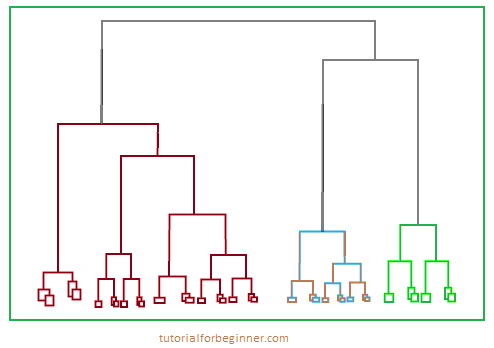## Fuzzy Clustering

A data object can belong to more than one group or cluster in fuzzy clustering, which is a sort of soft technique. Each dataset has a set of membership coefficients that are based on the degree of cluster membership. This sort of clustering is exemplified by the Fuzzy C-means algorithm, which is also known as the Fuzzy k-means algorithm.

## Clustering Algorithms

Clustering methods are classified according to their models, which are described above. Various clustering techniques have been described, but only a few are widely utilized. The clustering algorithm is determined by the type of data we're working with. Some algorithms, for example, must guess the number of clusters in a given dataset, while others must identify the shortest distance between the dataset's observations.

The following are some of the most extensively used Clustering algorithms in machine learning:

1. k-means algorithm: is one of the most commonly used clustering algorithms. It divides the samples into separate clusters with equal variances to classify the data. This approach requires the number of clusters to be provided. With O's linear complexity, it's quick and requires less computations (n).
2. Mean-shift algorithm: The mean-shift algorithm attempts to locate dense spots within a smooth distribution of data points. It is an example of a centroid-based model that updates candidates for centroid to be the center of points within a particular region.
3. DBSCAN Algorithm: Density-Based Spatial Clustering of Applications with Noise is the name of the DBSCAN algorithm. It's an example of a density-based model that's similar to the mean-shift, but with a few key differences. The high-density zones are separated from the low-density areas in this algorithm. As a result, the clusters might take on any shape.
4. Using GMM for Expectation-Maximization Clustering: This algorithm can be used as a replacement for the k-means algorithm or in situations when the k-means algorithm fails. The data points in GMM are supposed to be Gaussian distributed.
5. Agglomerative Hierarchical algorithm: Agglomerative Bottom-up hierarchical clustering is accomplished using the Agglomerative hierarchical algorithm. Each data point is initially regarded as a single cluster, and then the clusters are merged one by one. A tree-structure can be used to illustrate the cluster hierarchy.
6. Affinity Propagation: It differs from previous clustering methods in that it does not require the number of clusters to be specified. Each data point sends a message to the other data points until they all agree. It has an O(N^2T) time complexity, which is the algorithm's fundamental flaw.

## Applications of Clustering

The following are some well-known clustering applications in Machine Learning:

• In Identification of Cancer Cells: Clustering algorithms are commonly utilized for cancer cell identification. It splits the data sets into cancerous and non-cancerous groups.
• In Search Engines: Search engines use the clustering process as well. The search result is based on the object that is the most similar to the search term. It accomplishes this by putting related data objects in a separate group from the other dissimilar objects. The quality of the clustering algorithm utilized determines how accurate a query is.
• Customer segmentation: It is a technique used in market research to divide customers into groups based on their preferences and choices.
• In Biology: The image recognition technology is utilized in the biology stream to classify different species of plants and animals.
• In Land Use: In the GIS database, the clustering technique is utilized to discover areas with similar land use. This can be very beneficial in determining what purpose a specific piece of land should be used for, or which purpose it is better fit for.